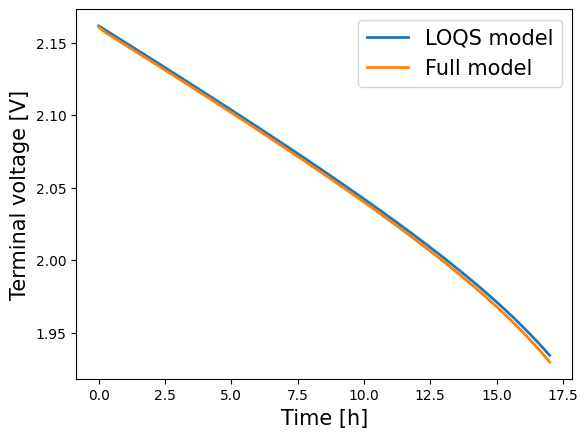Tip

An interactive online version of this notebook is available, which can be accessed viaWe compare a standard porous-electrode model for lead-acid batteries with two asymptotic reductions. For a more in-depth introduction to PyBaMM models, see the SPM notebook. Further details on the models can be found in .

:

%pip install "pybamm[plot,cite]" -q    # install PyBaMM if it is not installed
import pybamm
import numpy as np
import os
import matplotlib.pyplot as plt
os.chdir(pybamm.__path__+'/..')

WARNING: You are using pip version 22.0.4; however, version 22.3.1 is available.
You should consider upgrading via the '/home/mrobins/git/PyBaMM/env/bin/python -m pip install --upgrade pip' command.
Note: you may need to restart the kernel to use updated packages.


## “Full” model#

### Electrolyte Concentration#

$\begin{split}\frac{\partial }{\partial t}\left(\epsilon c\right) = -\frac{\partial N}{\partial x} + sj, \\ N = -\frac{\epsilon^b D(c)}{\mathcal{C}_\text{e}} \frac{\partial c}{\partial x}\\ N\big|_{x=0}= N\big|_{x=1}=0, \\ c\big|_{t=0} = 1\end{split}$

### Porosity#

$\begin{split}\frac{\partial \epsilon}{\partial t} = -\beta^\text{surf}j, \\ \epsilon\big|_{t=0} = \epsilon^0\end{split}$

### Electrolyte Current#

$\begin{split}\frac{\partial i_{\text{e}}}{\partial x} = j, \\ \mathcal{C}_\text{e} i_{\text{e}} = \epsilon_k^b \kappa(c) \left( \chi \frac{\partial}{\partial x}\log(c) - \frac{\partial\phi_{\text{e}}}{\partial x}\right)\\ i_{\text{e}}\big|_{x=0}= i_{\text{e}}\big|_{x=1}=0,\end{split}$

### Electrode Current#

$\begin{split}\frac{\partial i_{\text{s}}}{\partial x} = -j,\\ i_{\text{s}} = -\sigma\frac{\partial\phi_{\text{s}}}{\partial x},\\ \phi_{\text{s}}\big|_{x=0} = i_{\text{s}}\big|_{x=l_\text{n}} = i_{\text{s}}\big|_{x=1-l_\text{p}} = 0, \\ i_{\text{s}}\big|_{x=1}=\mathcal{I},\end{split}$

### Interfacial current density#

$\begin{split}j = \begin{cases} 2j_0(c) \sinh\left(\eta\right), \quad &0 < x < l_\text{n} \\ 0, \quad &l_\text{n} < x < 1-l_\text{p} \\ 2j_0(c) \sinh\left(\eta\right), \quad &1-l_\text{p} < x < 1 \end{cases} \\ \eta = \phi_{\text{s}} - \phi_\text{e} - U(c),\end{split}$

This model is implemented in PyBaMM as the Full model

:

full = pybamm.lead_acid.Full()


$\begin{split}\frac{\mathrm{d} }{\mathrm{d} t}\left(\epsilon c\right) = (s_\text{n} - s_\text{p})\mathrm{I}, \\ \frac{\mathrm{d} \epsilon}{\mathrm{d} t} = -\beta^\text{surf}j, \\ j = \begin{cases} \mathrm{I}/l_\text{n}, \quad &0 < x < l_\text{n} \\ 0, \quad &l_\text{n} < x < 1-l_\text{p} \\ -\mathrm{I}/l_\text{p}, \quad &1-l_\text{p} < x < 1 \end{cases} \\ \phi_\text{e} = -U_\text{n}(c) + \sinh^{-1}\left(\frac{\mathrm{I}}{2l_\text{n}j_{0\text{n}}(c)}\right) \\ V = -\phi_\text{e} + U_\text{p}(c) - \sinh^{-1}\left(\frac{\mathrm{I}}{2l_\text{p}j_{0\text{p}}(c)}\right) \\\end{split}$

This model is implemented in PyBaMM as LOQS (leading-order quasi-static)

:

loqs = pybamm.lead_acid.LOQS()


## Solving the models#

We load process parameters for each model, using the same set of (default) parameters for all. In anticipation of changing the current later, we make current an input parameter

:

# load models
models = [loqs, full]

# process parameters
param = models.default_parameter_values
param["Current function [A]"] = "[input]"
for model in models:
param.process_model(model)


Then, we discretise the models, using the default settings

:

for model in models:
# load and process default geometry
geometry = model.default_geometry
param.process_geometry(geometry)

# discretise using default settings
mesh = pybamm.Mesh(geometry, model.default_submesh_types, model.default_var_pts)
disc = pybamm.Discretisation(mesh, model.default_spatial_methods)
disc.process_model(model)


Finally, we solve each model using CasADi’s solver and a current of 1A

:

timer = pybamm.Timer()
solutions = {}
t_eval = np.linspace(0, 3600 * 17, 100) # time in seconds
for model in models:
timer.reset()
solution = solver.solve(model, t_eval, inputs={"Current function [A]": 1})
print("Solved the {} in {}".format(model.name, timer.time()))
solutions[model] = solution

Solved the LOQS model in 64.611 ms
Solved the Full model in 736.504 ms


## Results#

To plot the results, the variables are extracted from the solutions dictionary. For example, we can compare the voltages:

:

for model in models:
time = solutions[model]["Time [h]"].entries
voltage = solutions[model]["Voltage [V]"].entries
plt.plot(time, voltage, lw=2, label=model.name)
plt.xlabel("Time [h]", fontsize=15)
plt.ylabel("Voltage [V]", fontsize=15)
plt.legend(fontsize=15)
plt.show()Alternatively, using QuickPlot, we can compare the values of some variables

:

solution_values = [solutions[model] for model in models]
quick_plot = pybamm.QuickPlot(solution_values)
quick_plot.dynamic_plot();


If we update the current, setting it to be 20 A, we observe a greater discrepancy between the full model and the reduced-order models.

:

t_eval = np.linspace(0, 3600, 100)
for model in models:

At t = 0.0087774 and h = 1.94278e-62, the corrector convergence failed repeatedly or with |h| = hmin.